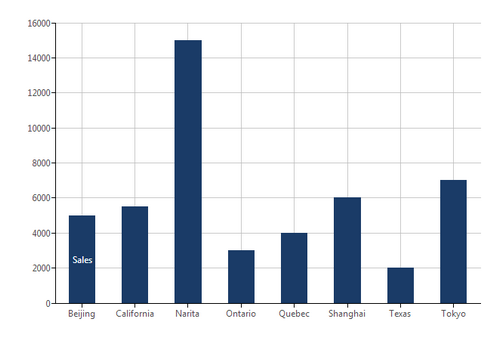# Totals (SUM)

Contents[Hide]

The Sum function calculates the total sum for any number of input value series.

## 1. Syntax

```SUM(d0,Alignments...)
```

## 2. Input

The Sum function requires the following input:

• d0 - The set of data values for which the Sum is calculated.Formula - Sum: Input data

## 3. Parameters

The Sum function requires the following parameters:

• Alignments... (One or More, Optional) – Hierarchy placeholders to be used as the alignment axis.

## 4. Output

The Sum function generates the following output:

• Total - A single value representing the total sum of the input values.

### 4.1. Change the chart type (optional)

For example, changing the chart type of the series derived from the formula from a Bar Chart to a Line Chart.

1. Click the series, right click, and select Re-visualize.

2. Select the new chart type.

To display the output in a separate control, such as a Data Label, you can use a formula visualization.